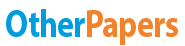# Zinc Titration with Edta

Essay by   •  March 5, 2012  •  Lab Report  •  515 Words (3 Pages)  •  2,826 Views

## Essay Preview: Zinc Titration with Edta

Report this essay
Page 1 of 3

Zinc Titration with EDTA

Abstract

The initial stages of the lab required the standardizations of ehylendiamineteraacetic acid by utilizing calcium carbonate solution containing CaCO3, water and 1:1 HCL acid as a titrant. Eriochrom Black T was added to the EDTA solution as an indicator in which turns from wine red to blue when an endpoint is reached. Three zinc lozenges in which were dissolved in a buffer solution maintaining a PH of 5.5, were then titrated with EDTA solution. The indicator Xylenol orange was added to the solution as it provided a definitive color change from pink to yellow when an endpoint was reached. When evaluating the results it was conclusively determined that the zinc lozenges within each trial contained 12.77 mg, 12.92 mg, and 13.06 mg of zinc. The percent average of zinc in each lozenge was calculated as 0.9246% Zn/Lozenge.

Data and Results

Table 1.1 Standardization of EDTA Solutions

Trial 1 Trial 2 Trial 3

Final Volume of EDTA in Burette (mL) 29.1 34.2 19.4

Initial Volume of EDTA in Burette (mL) 43.8 48.8 34.2

Total Amount of Titrant used (mL) 14.7 14.2 14.8

Amount of CaCO3 Reacted (g) 1.60x10^-2 1.60x10^-2 1.60x10^-2

Table 1.2 Calculated Values for Standardization

Chemical Amount

Mass of CaCO3 (g) 0.2000 g

Moles of CaCO3 (mol) 1.60x10^-4

Molarity of CaCO3 (mol/L) 7.993 x 10^-3

Moles of EDTA (mol) 1.60x10^-4

Molarity of EDTA in Trial 1 (mol/L) 1.087 x 10^-2

Molarity of EDTA in Trial 2 (mol/L) 1.13 x 10^-2

Molarity of EDTA in Trial 3 (mol/L) 1.08 x 10^-2

Average Molarity of EDTA (mol/L) 1.098 x 10^-2

Standard Deviation 2.45 x 10^-4

Relative Standard Deviation (%) 2.232

Table 1.3 Volumes Calculated for the Titration of Zinc

Trial 1 Trial 2 Trial 3

Final Volume (mL) 30.7 30.7 42.4

Initial Volume (mL) 48.5 12.7 24.2

Amount of Titrant utilized (mL) 17.8 18 18.2

Table 1.4 Calculated Standard Deviation, Percent Averages, and Relative Standard Deviation of Zinc

Calculated Amount

...

...